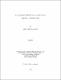## Analysis of low voltage regulator efficiency based on ferrite inductor2010
##### Publisher
University of Alabama Libraries
##### Abstract

Low voltage regulator based on ferrite inductor, using single- and two-phase topologies, were designed and simulated in MATLAB. Simulated values of output voltage and current were used to evaluate the buck converter (i.e., low voltage regulator) for power efficiency and percentage ripple reduction at frequencies between 1 and 10 MHz with variable loads from 0.024 to 4 ohms. The parameters, such as inductance of 20 nH, quality factor of 15 of fabricated ferrite inductor and DC resistance (DCR) of 8.3 milli ohms, were used for efficiency analysis of the converter. High current around 40 A was achieved by the converter at low load values. Low output voltage in the range of 0.8-1.2 V was achieved. The simulated results for the single- and two-phase converter were compared for maximum efficiency and lowest ripple in output voltage and current. The maximum efficiency of 97 % with load of 0.33 ohms and the lowest ripple current of about 2.3 mA were estimated for the two-phase converter at 10 MHz. In summary, the two-phase converter showed higher efficiency and lower ripple voltage and current than those of the single-phase converter. In addition, the efficiency of single- and two-phase converters based on ferrite inductor was compared to single- and two-phase converters based on air-core inductor. It was found that the power efficiency of the two-phase converter using ferrite inductor was 10 % higher than the converter using air-core inductor at 10 MHz with a load of 0.024 ohms.

##### Description
Electronic Thesis or Dissertation
##### Keywords
Engineering, Electronics and Electrical